# Question:How do I speed up the Evaluation of Elliptical functions?

## Question:How do I speed up the Evaluation of Elliptical functions?

Maple 2016

Question:- can the procedure given below called "epi" be speeded up by compiling/ using evhf.If so how? My paple code is at the bottom.

First some background information.

Recently I ran into a difference in usage of a couple of elliptical functions between Maple and Mathematica.  This first case concerned EllipticalPi. The author of the blog kindly wrote  a Maple procedure to produce the same results as Mathematica’s  usage of ElllipticalPi.

I tested the basic integral that produces the EllipticPi    Ell := int(1/(1-nu*JacobiSN(t, k)^2), t)  answer      Ell := EllipticPi(JacobiSN(t, k), nu, k). They do not produce the same outcome. Plots are in the document .  They agree in one quarter only.

I then ran into a difference in usage of  EllipticF. This time I was able to get to same outcome myself using Maple’s help.

“It is worth noting the difference between the Legendre normal form of the Incomplete Elliptic integral of the first kind (see A&S 17.2.7), in Maple represented by EllipticF(z,k) but for the splitting of the square root in the denominator of the integrand (see definition lines above), and the normal trigonometric form of this elliptic integral (see A&S 17.2.6), in Maple represented by the InverseJacobiAM function
InverseJacobiAM(phi,k);

That worked fine.

There is no mention in the help for usage implementation of EllipticPi as opposed to different usages as there is with EllipticF. I do not know if there is a way in Maple of achieving the same enactment as Mathematica in this case, without the Procedure I  was  given.

Elliptic Pi in Mathematica and Maple

We use EllipticPi when we write exact solutions of rotation of a free asymmetric top. While solving Euler’s equations for angular velocity or angular momentum in the body frame we need Jacobi elliptic functions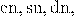solving the differential equation for the attitude matrix involves EllipticPi function. As I have explained it in Taming the T-handle continued we need the integral

(1)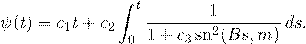In Mathematica this is easily implemented as

(2)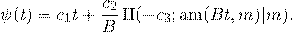However, as pointed out by Rowan in a comment to Taming the T-handle continued , the same formula does not work with Maple.

While the documentations of both Mathematica and Maple contain links to Abramowitz and Stegun Handbook of Mathematical Functions, they use different definitions. Here is what concerns us, from p. 590 of the 10th printing: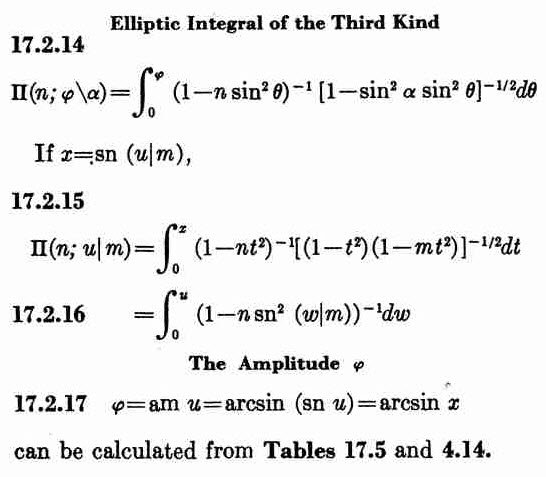What we need is 17.2.16, while Maple is using 17.2.14. To convert we need to set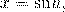but such a conversion is possible only in the domain where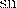can be inverted. We can do it easily for sufficiently small values of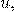but not necessarily for values that contain several quarter-periods.

The following Maple procedure does the job:

epi := proc (t::float, nu::float, k::float) local t2, n, dt, ep0, res; ep0 := EllipticPi(nu, k); t2 := EllipticK(k); n := floor(t/t2); dt := t-t2*n; if type(n, even) then res := Re(n*ep0+EllipticPi(JacobiSN(dt, k), nu, k)) else res := Re((1+n)*ep0-EllipticPi(JacobiSN(t2-dt, k), nu, k)) end if end proc

HAs an example here is the Maple plot for nu=-3, k=0.9:
plot(('epi')(t, -3.0, .9), t = -20 .. 20)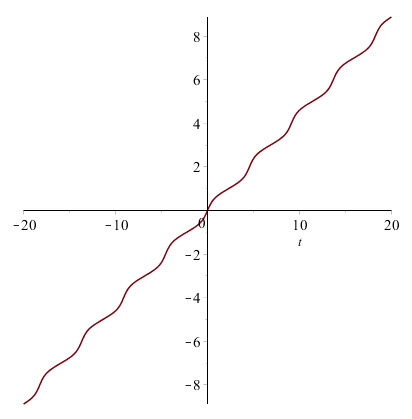And here is the corresponding Mathematica plot: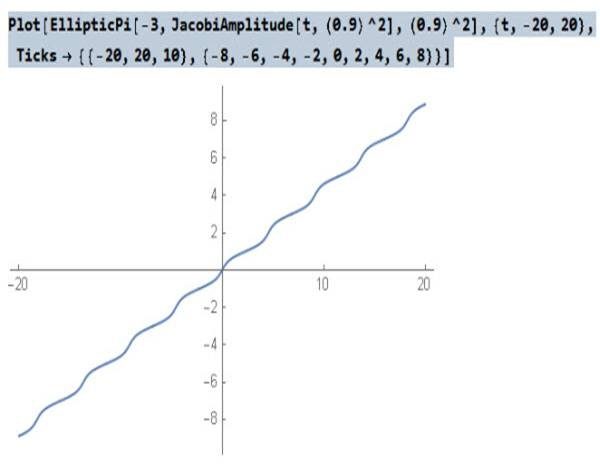The function epi(t,nu, k) defined above for Maple gives now the same result as EllipticPi(nu,JacobiAM(t,k^2),k^2) in Mathematica.

restart;
epi := proc (t, nu, k) local t2, n, dt, ep0, res; ep0 := EllipticPi(nu, k); t2 := EllipticK(k); n := floor(t/t2); dt := t-t2*n; if type(n, even) then res := Re(n*ep0+EllipticPi(JacobiSN(dt, k), nu, k)) else res := Re((1+n)*ep0-EllipticPi(JacobiSN(t2-dt, k), nu, k)) end if end proc;

Ell := int(1/(1-nu*JacobiSN(t, k)^2), t);
Ell := EllipticPi(JacobiSN(t, k), nu, k)
k := .9;
k := 0.9
nu := -3;
nu := -3
plot([epi(t, nu, k), Ell], t = -8 .. 20);﻿